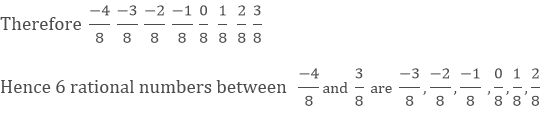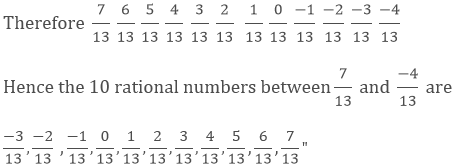# Chapter 5: Operations on Rational Numbers Exercise – 5.5

### Question: 1

Find six rational numbers between (-4)/8 and 3/8

### Solution:

We know that

- 4, -3, -2, -1, 0, 1, 2, 3### Question: 2

Find 10 rational numbers between 7/13 and (- 4)/13

### Solution:

We know that

76543210 -1 -2 -3 –4### Question: 3

State true or false:

(i) Between any two distinct integers there is always an integer.

(ii) Between any two distinct rational numbers there is always a rational number.

(iii) Between any two distinct rational numbers there are infinitely many rational numbers.

(i) False
(ii) True
(iii) True